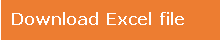# Get Sheet Names

How To Get Sheet Names Using VBA in Microsoft Excel

In case you want to find out a way which can get you all the names of the sheet that are visible i.e. not hidden.

In this article, we will learn how to get names of the visible sheets only, using VBA code.

Question): I have multiple sheets in one file & I have hidden the sheets which I do not want others to see; I want a code that will give me the name of all the visible sheets.

Let us consider we have 5 sheets & we intentionally hide a particular sheet.

To get name of the visible sheets, we need to follow the below steps:

• Click on Developer tab
• From Code group, select Visual Basic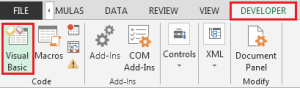Copy the below code in the standard module

Sub VisibleSheets()

Dim i As Integer, j As Integer: j = 1

Cells(1, 1).CurrentRegion.Cells.Clear

For i = 1 To Sheets.Count

If Sheets(i).Visible = -1 Then

Cells(j, 1) = Sheets(i).Name

j = j + 1

End If

Next

End Sub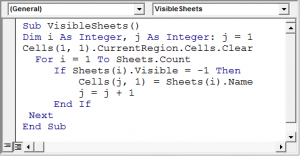• First time when you run the code, you will get the names of all the sheets in current sheet in column A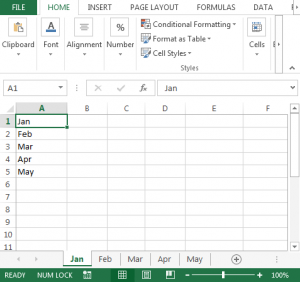• If we hide Jan sheet then we will have following list of sheet names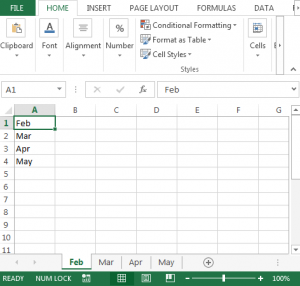In this way, we can get the name of all the visible sheets, using vba code.